﻿ 基于可变局部边缘模式的绿色植物物种识别
«上一篇文章快速检索 高级检索

 智能系统学报2018, Vol. 13Issue (4): 571-576  DOI: 10.11992/tis.2017090240

### 引用本文CHEN Xiaomeng, WANG Yu, XIAO Hongbing. Identification of green plant species based on varied local edge patterns[J]. CAAI Transactions on Intelligent Systems, 2018, 13(4), 571-576. DOI: 10.11992/tis.201709024.### 文章历史

Identification of green plant species based on varied local edge patterns
CHEN Xiaomeng, WANG Yu, XIAO Hongbing
School of Computer and Information Engineering, Beijing Technology and Business University, Beijing 100048, China
Abstract: The identification of green plant species has the potential for important applications and economic value in many respects, such as protection of the environmental ecology and preparation of traditional Chinese medicine, as well as agricultural and horticultural applications. The edge of an object is an intuitive, simple, and effective object recognition feature. To overcome the shortcomings of traditional edge operators, such as few directions, single scale, and inflexible operation, in this paper, we propose a green-plant-species recognition algorithm based on circular local edge patterns, which has multiple scales and multiple directional features. We conducted a series of experiments to consider threshold subdivisions in the self-built green-plant-species database. The results show that the proposed algorithm effectively complements the edge information that is lost by the fewer edge directions and single scale of traditional edge operators, and can be effectively applied to identify green plant species.
Key words: varied local edge patterns    extraction of edge feature    identification of plant species

1 VLEP算子

VLEP算子是一种灵活的圆形可变局部边缘模式算子，用 ${\rm{VLEP}}_{P,R}^\theta$ 表示， $P$ 为近邻点个数， $R$ 为半径， $\theta$ 表示边缘方向。该算子包括两大类，一类是局部边缘模式算子，另一类是局部非边缘模式算子。

1.1 局部边缘模式算子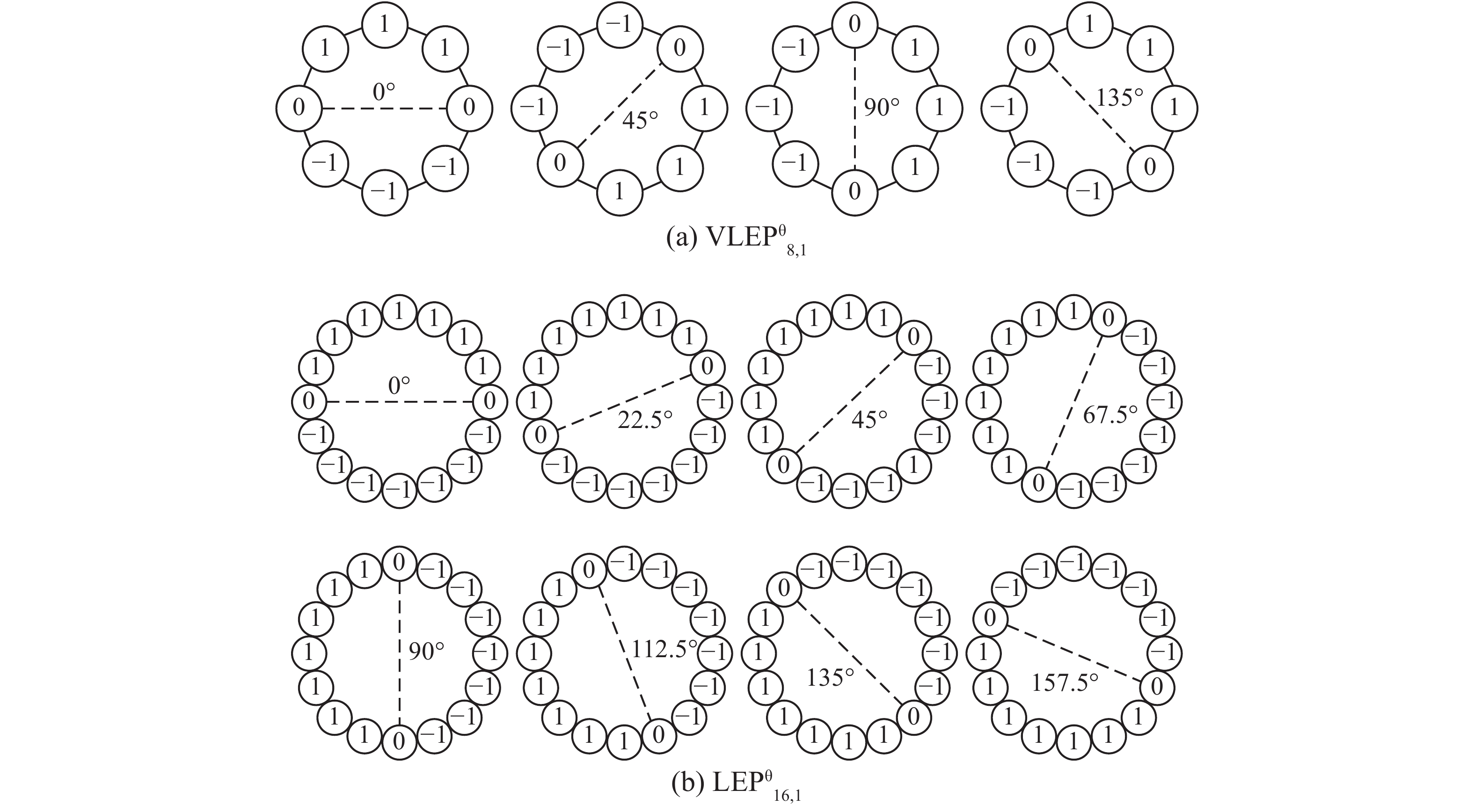Download: 图 1 两组局部边缘模式算子 Fig. 1 Two groups of local edge pattern operators
1.2 局部非边缘模式算子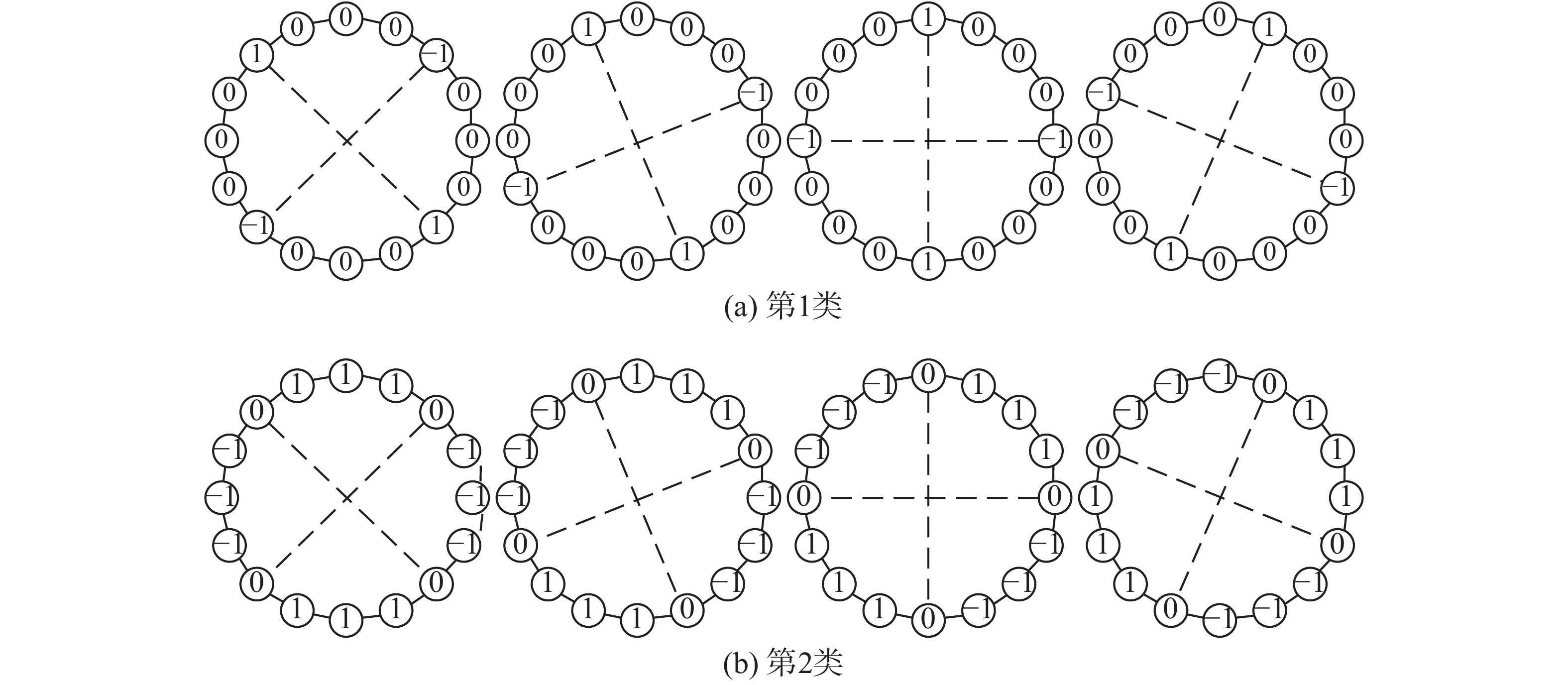Download: 图 2 一组局部非边缘模式算子 ${\rm{VLEP}}_{16,R}^\theta$ Fig. 2 A group of local non-edge pattern operators ${\rm{VLEP}}_{16,R}^\theta$
1.3 VLEP算子的多尺度与多方向属性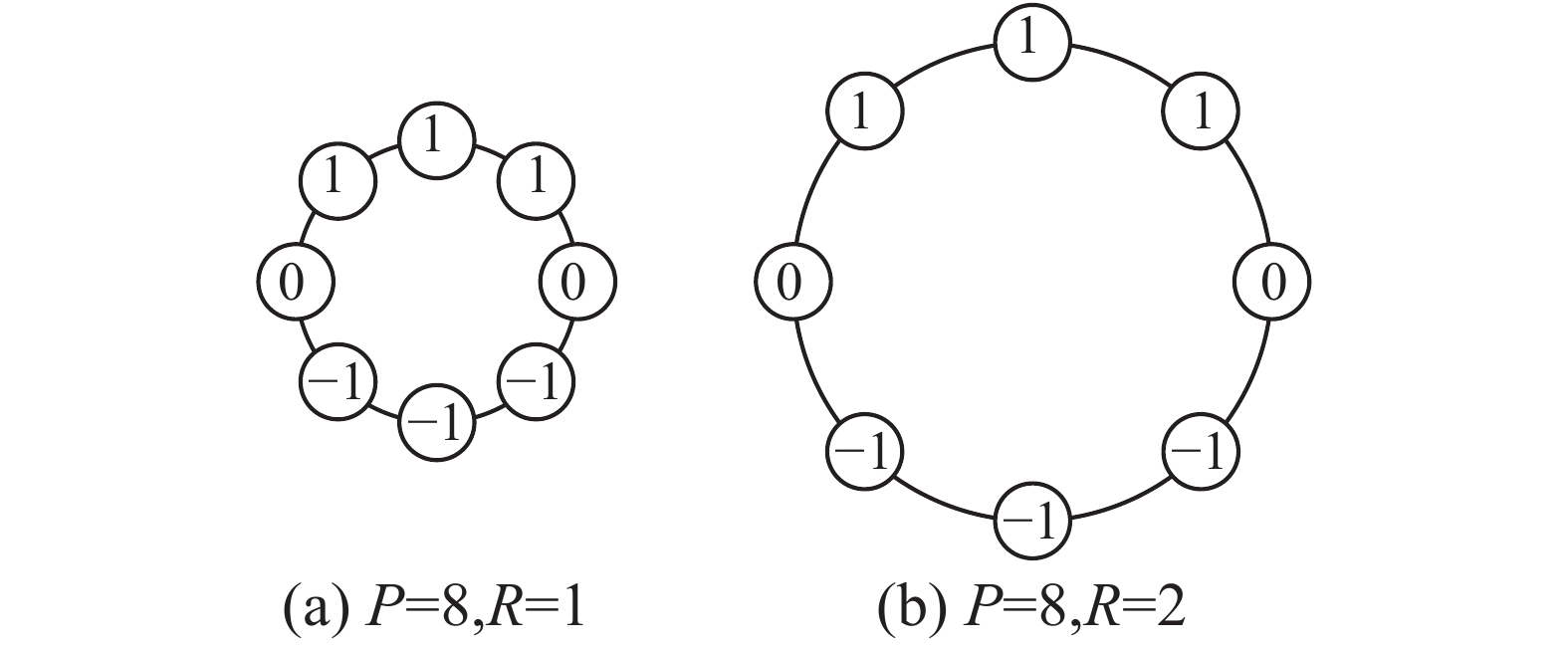Download: 图 3 不同尺度VLEP算子实例 Fig. 3 An example of multi-scale VLEP operators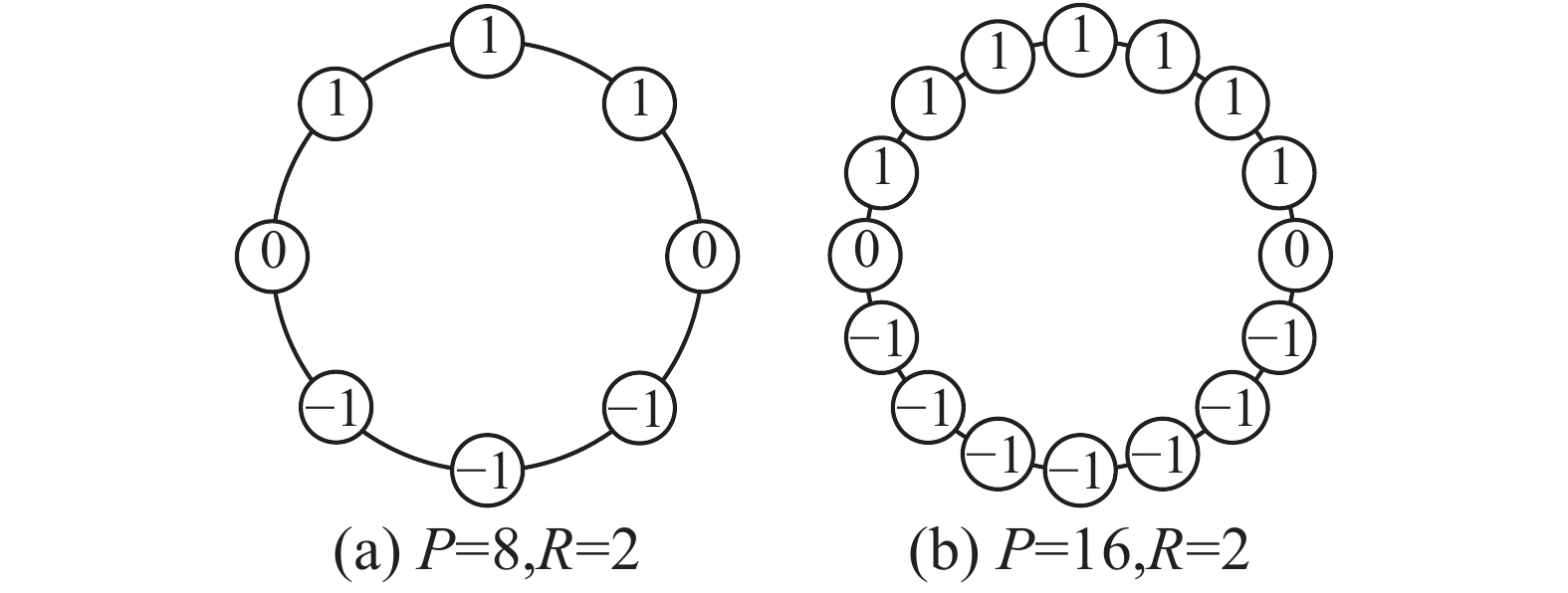Download: 图 4 不同方向(分辨率)VLEP算子实例 Fig. 4 An example of multi-direction (or multi-resolution) VLEP operators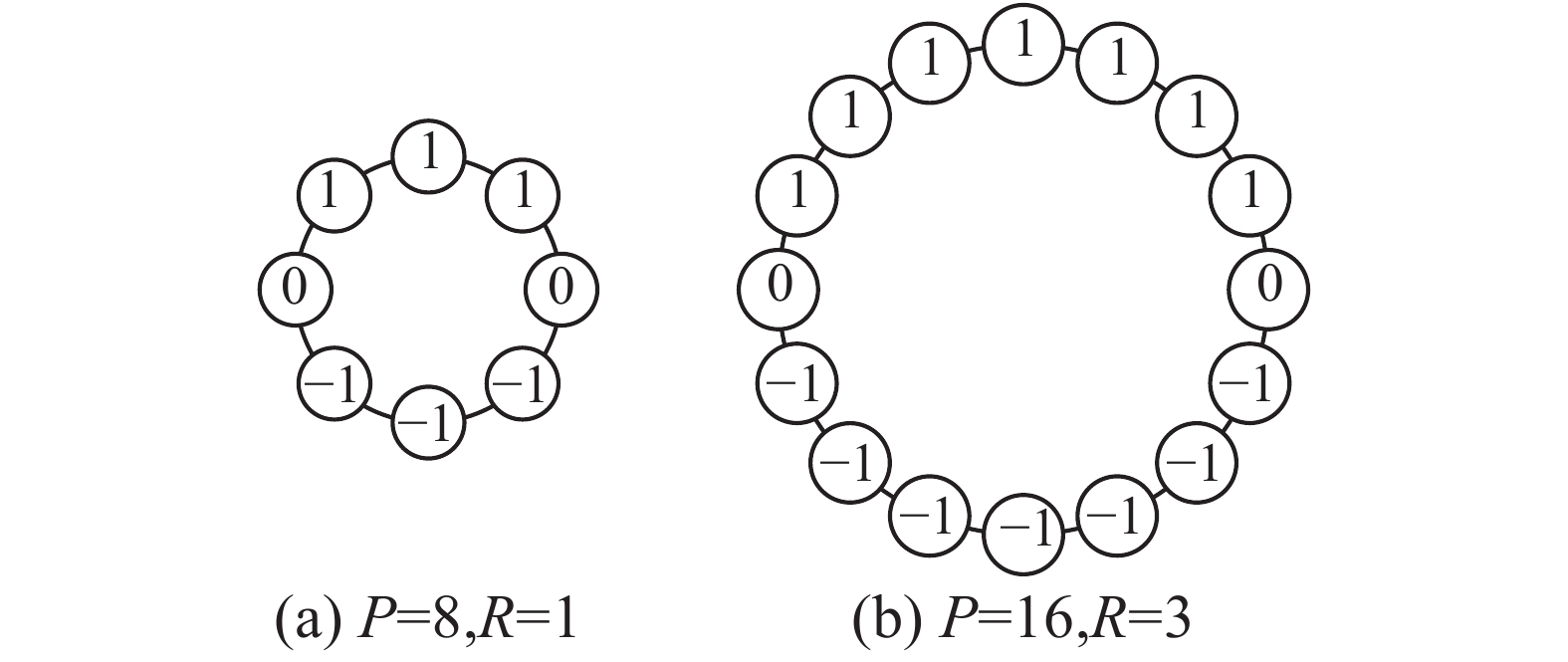Download: 图 5 不同尺度与不同方向(分辨率)VLEP算子实例 Fig. 5 An example of multi-scale and multi-resolution VLEP operators
2 基于VLEP算子的边缘特征提取

 $G_{P,R}^\theta (x,y) = {\rm{VLEP}}_{P,R}^\theta \otimes I(x,y)$ (1)

 $E_{P,R}^\varphi = \max (|G_{P,R}^\theta |) , \; \varphi \in \theta$ (2)

 $T_{P,R}^\varphi = \frac{{\displaystyle\sum\limits_{x = 0}^{M - 1} {\displaystyle\sum\limits_{y = 0}^{N - 1} {f(x,y)} } }}{{M \times N}}$ (3)

$(x,y)$ $M \times N$ 维图像 $I(x,y)$ 的像素点。然后，可以得到一个特征直方图 ${H_{P,R}}$ ，包括不同的边缘或非边缘模式出现的概率频次。

 ${{{H}}_{P,R}} = (T_{P,R}^{{\varphi _1}}, \cdots ,T_{P,R}^{{\varphi _K}}, \cdots ,T_{P,R}^{{\varphi _m}})$ (4)

3 边缘特征细分

 ${V_{\rm{th}}} = E_{P,R}^\varphi (\frac{N}{B} \times w + 1), \; (w = 1, 2, \cdots ,B - 1)$ (5)

 $\lambda = B\times(\frac{P}{2} + \frac{P}{4}\times A)$ (6)

 ${{H}}_{P,R}' = (T_{P,R}^{{\varphi _{11}}}, \cdots \! ,T_{P,R}^{{\varphi _{1B}}}, \cdots \! T_{P,R}^{{\varphi _{k1}}}, \cdots \! ,T_{P,R}^{{\varphi _{kB}}}, \cdots \! ,T_{P,R}^{{\varphi _{m1}}}, \cdots \! ,T_{P,R}^{{\varphi _{mB}}})$ (7)
4 分类器

 $D\left( {\overline {{{H}}_k'} ,{{H}}_{\rm{test}}'} \right) = \sqrt {\left( {\overline {{{H}}_k'} - {{H}}_{\rm{test}}'} \right){S^{ - 1}}{{\left( {\overline {{{H}}_k'} - {{H}}_{\rm{test}}'} \right)}^{\rm{T}}}}$ (8)

5 实验结果与分析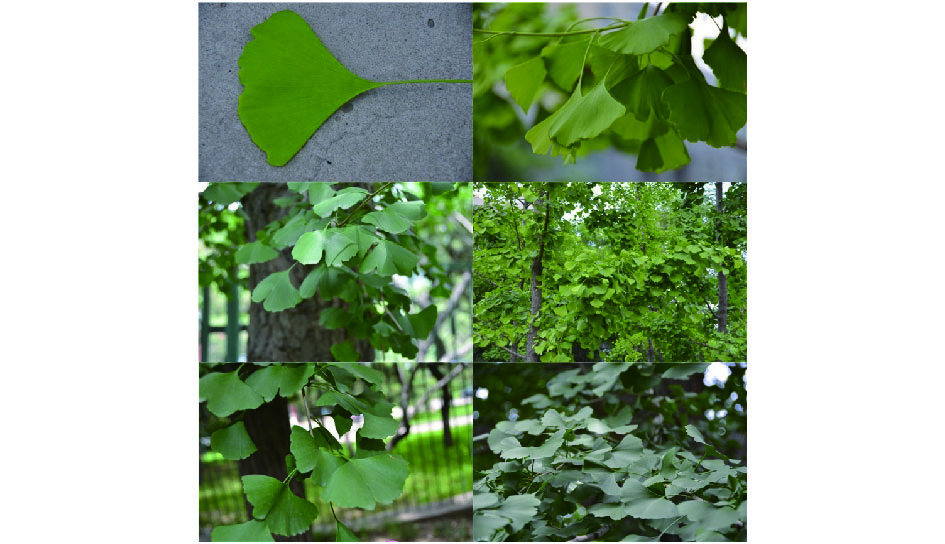Download: 图 6 绿色植物物种数据库图例(银杏树) Fig. 6 Some examples of the green plants database (ginkgo)
5.1 相同阈值下不同半径和近邻点VLEP实验结果表 1 相同阈值数下的 ${\rm{VLEP}}_{P,R}^\theta$ 算法识别率 Tab.1 Recognition results of ${\rm{VLEP}}_{P,R}^\theta$ with the same thresholds　　　　　　　　　　　　　　　　%

5.2 阈值细分的作用表 2 不同阈值数下的 ${\rm{VLEP}}_{P,R}^\theta$ 算法识别率 Tab.2 Recognition results of ${\rm{VLEP}}_{P,R}^\theta$ with different thresholds　　　　　　　　　 　　　　　%

5.3 与传统边缘算子的对比实验表 3 对比算法识别率 Tab.3 Recognition results of contrast algorithms

6 结束语

  胡秋萍. 基于叶片形状特征的植物识别技术研究[D]. 西安: 西安电子科技大学, 2014. HU Qiuping. The study of plant identification technology based on leaves’ shape features[D]. Xi’an: Xidian University, 2014. (0)  叶绪伦, 赵杰煜, 陈能仑. 结合LDA主题模型的植物叶片形状描述及分类[J]. 模式识别与人工智能, 2016, 29(3): 263-271. YE Xulun, ZHAO Jieyu, CHEN Nenglun. Leaf shape description and classification with LDA topic mode[J]. Pattern recognition and artificial intelligence, 2016, 29(3): 263-271. (0)  刘骥, 曹凤莲, 甘林昊. 基于叶片形状特征的植物识别方法[J]. 计算机应用, 2016, 36(s2): 200-202. LIU Ji, CAO Fenglian, GAN Linhao. Plant identification method based on leaf shape features[J]. Journal of computer applications, 2016, 36(s2): 200-202. (0)  SHRIVAKSHAN G T, CHANDRASEKAR C. A comparison of various edge detection techniques used in image processing[J]. International journal of computer science issues, 2012, 9(5): 269-276. (0)  KAUR K, MALHOTRA S. A survey on edge detection using different techniques[J]. International journal of application or innovation in engineering and management, 2013, 2(4): 496-500. (0)  ONN U, SINGLA P. A survey on conventional edge detection techniques[J]. Journal of algebra, 2014, 397: 1-17. DOI:10.1016/j.jalgebra.2013.08.022 (0)  BERZINS V. Accuracy of Laplacian edge detectors[J]. Computer vision, graphics, and image processing, 1984, 27(2): 195-210. DOI:10.1016/S0734-189X(84)80043-2 (0)  DUDA R O, HART P E. Pattern classification and scene analysis[M]. New York: Wiley, 1973. (0)  MAINI R, SOHAL J S. Performance evaluation of Prewitt edge detector for noisy images[J]. GVIP journal, 2006, 6(3): 39-46. (0)  OGAWA K, ITO Y, NAKANO K. Efficient Canny edge detection using a GPU[C]//2010 First International Conference on Networking and Computing. Hangzhou, China, 2010: 279–280. (0)  VOLLMER J, MENCL R, MÜLLER H. Improved Laplacian smoothing of noisy surface meshes[J]. Computer graphics forum, 2010, 18(3): 131-138. (0)  HUSSEIN W B, MOATY A A, HUSSEIN M A, et al. A novel edge detection method with application to the fat content prediction in marbled meat[J]. Pattern recognition, 2011, 44(12): 2959-2970. DOI:10.1016/j.patcog.2011.04.028 (0)  DI Haibin, GAO Dengliang. Gray-level transformation and Canny edge detection for 3D seismic discontinuity enhancement[J]. Computers and geosciences, 2014, 72: 192-200. DOI:10.1016/j.cageo.2014.07.011 (0)  GAO Wenshuo, ZHANG Xiaoguang, YANG Lei, et al. An improved Sobel edge detection operator[C]//2010 3rd IEEE International Conference on Computer Science and Information Technology. Chengdu, China, 2010: 67–71. (0)  WANG Yu, ZHAO Yongsheng, CAI Qiang, et al. A varied local edge pattern descriptor and its application to texture classification[J]. Journal of visual communication and image representation, 2016, 34: 108-117. DOI:10.1016/j.jvcir.2015.11.001 (0)